Interferometer Webapps and Experiment Simulators.

1)  Interactive Michelson Interferometer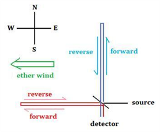A webapp that allows one to simulate the Michelson and Morley experiment and set a variety of conditions to determine what amount of fringe shift would be detected when the device is rotated from 0 to 90 degrees. Variables include Lorentz contraction, refractive index, relative speed of frame (ether wind), path length, and wavelength.  Uses equations developed in "The Premise for Comparing Ether Theory to Relativity Theory" in the Theory and Experimental Tests section.

2) Interactive Hoek Interferometer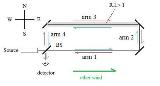A webapp that allows one to simulate the Hoek experiment and set a variety of conditions to determine what amount of fringe shift would be detected when the device is rotated from 0 to 90 degrees. Variables include Lorentz contraction, refractive index, relative speed of frame (ether wind), path length, and wavelength.  Uses equations developed in "The Premise for Comparing Ether Theory to Relativity Theory" in the Theory and Experimental Tests section.

3) Interactive Michelson-Gale Interferometer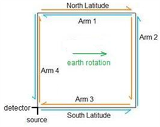A webapp that allows one to simulate the Michelson-Gale experiment. The original parameters are pre-loaded. The dimensions of the experiment, the latitude, the light wavelength etc. can be adjusted to see they effect the experimental outcome.

4A) Interactive Simple Sagnac Interferometer /Fibre-Optic Gyroscope (FOG)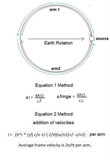A webapp that allows one to simulate the Sagnac effect. Shows how the propagation time for each beam on the rotating platform can be calculated using simple Galilean addition of velocities, and how in the frame of the rotating observer the velocity of each beam differs by the rotation velocity.

4B) Interactive Complex Sagnac Interferometer /Fibre-Optic Gyroscope (FOG)A webapp that allows one to simulate the Sagnac effect. The original parameters of the Michelson-Gale experiment are pre-loaded. Various parameters can be adjusted to see how rotation, translation, and the movement of the earth affect the readings of the interferometer.

5) Interactive Hafele and Keating Time Dilation Experiment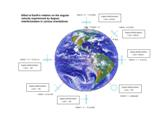A webapp to simulate the around the world time dilation experiment using   clocks flown on planes westbound and eastbound. The time lost or gained on each clock it determined with respect to a clock that remains on the ground at the starting point. The simulator can vary parameters such as the altitudes the clocks are flown at, the latitude of the path over the surface of the earth, and the total trip time for each flight. The individual terms (velocity, Sagnac and height) are calculated and then shown separately and as a net time gain/loss by each clock.

6)  Interactive Gravitational Potential and Coordinate Time Increment on Earth's Oblate Geoid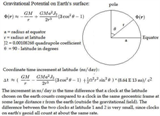A webapp to simulate the textbook calculation of gravitational potential on the earth's surface, and the coordinate time increment of clocks at different latitudes at sea level.

7)  Interactive Gravitational Potential and Coordinate Time Increment on Earth's Oblate GeoidA more advanced webapp simulation also comparing time dilation effects on moving clocks at velocities and heights over the earth's surface, similar to the Hafele and Keating experiment.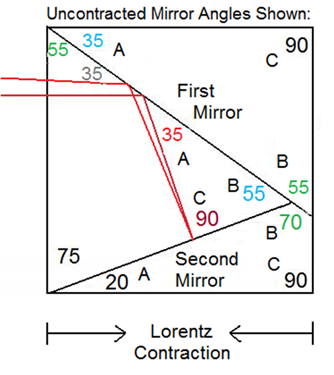A webapp that allows one to simulate the Esclangon Experiment.  The webapp allows one to adjust the mirror angles and hypothetical ether wind speed.  The vertical image displacement in the telescope on rotation is then calculated assuming that the mirrors would experience a Lorentz contraction in the direction of the ether wind. See article 5) in the "Our Experiments and Videos" section for additional details.

9) One Laser One-Way Speed of Light Interferometer Simulator.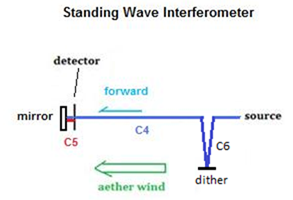A webapp that allows one to simulate a one-way speed of light experiment using one laser. This demonstrates how the fringe shift that arises due to a standing wave moving with respect to a sensor in an interferometer that is rotated through an aether wind will be cancelled by the frequency shift in the laser clock due to a mechanical time dilation effect predicted by Lorentz.

10) Two Laser One-Way Speed of Light Interferometer Simulator.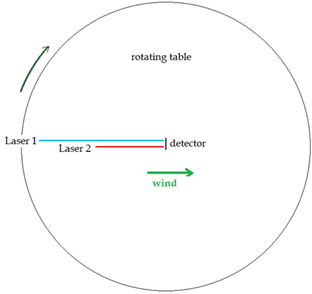A webapp that allows one to simulate a one-way speed of light experiment using two lasers. This demonstrates how the fringe shift that should arise due to differening path lengths and the laser beams crossing from parallel to perpendicular to an aether wind, will be cancelled by the frequency shift in the laser clock due to a mechanical time dilation effect predicted by Lorentz.

11) Local Time to Sidereal Time ConverterA webapp that allows one to calculate the sidereal time value for any local time value, knowing the date, local time, and longitude of the observer. Also calcuates the Julian date.

12) Shamir and Fox Experiment SimulatorA webapp demonstrating how refractive index has no effect on the measured speed of light in a Michelson interferometer. This simulator also breaks out the apparent time dilation along each arm, showing how it deviates from the average time dilation at the detector.

13) Kennedy Thorndike Experiment SimulatorA webapp demonstrating how orientation with respect to sidereal space and arm length has no effect on the measured speed of light in a Michelson interferometer. This simulator also breaks out the apparent time dilation along each arm, showing how it deviates from the average time dilation at the detector. Time dilation is shown to be not just a velocity effect but also an effect which depends on orientation with respect to velocity.

14) Rotating Triangle InterferometerA webapp demonstrating how light beams counter-travelling around a closed path will always show the same measured speed of light even if the interferometer is moving at a relatively high velocity through space. Includes a number of adjustable parameters

15) GPS Propagation Range Simulator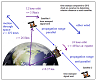A webapp demonstrating why it is that our high speed translational motion through space is never detected by GPS range measurements, even though the much smaller rotational velocity of the earth is easily detected via the Sagnac effect. In explaining this effect via apparent time dilation of the GPS transmitter, the necessity for trying to explain this issue using a dragged ether hypothesis becomes unnecessary.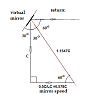We attempt to show why terrestrial aberration does not occur in a Michelson interferometer. The light beams are shown to arrive at the interferometer detector perfectly parallel despite any velocity, due to a physical Lorentz contraction of the apparatus and the beamsplitter.